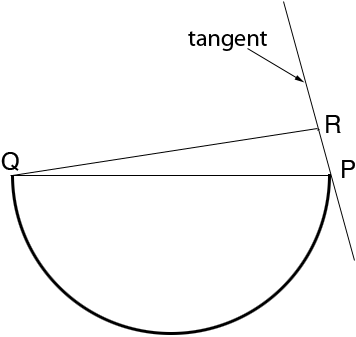SEARCH HOMEMath Central Quandaries & QueriesQuestion from elizabeth, a student: show that the radius of a circle meets a tangent line to the circle in a 90 degree angle. hint: start by assuming they are not perpendicular and at a contradiction.Hi Elizabeth,

Suppose that PQ is a diameter of a circle and construct the tangent line to the circle at P. Construct a line from Q to meet the tangent line at R.If the tangent line does not make a right angle with the diameter QP then P and R are different points. Since QP is a diagonal to the circle and angle PRQ is a right angle, what can you say about the point R?

PennyMath Central is supported by the University of Regina and The Pacific Institute for the Mathematical Sciences.